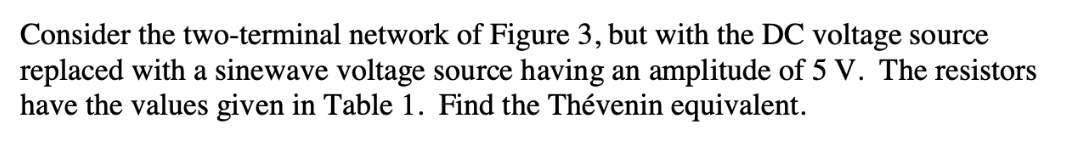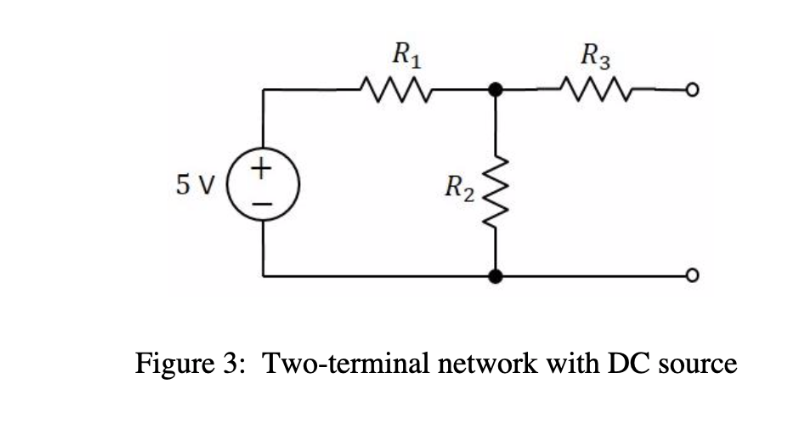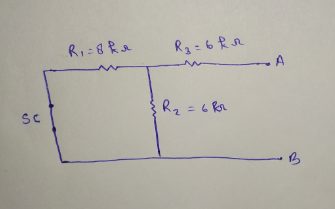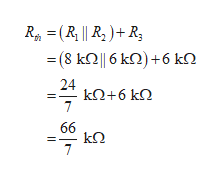# Consider the two-terminal network of Figure 3, but with the DC voltage sourcereplaced with a sinewave voltage source having an amplitude of 5 V. The resistorshave the values given in Table 1. Find the Thévenin equivalent R3R25 VFigure 3: Two-terminal network with DC source

Question
18 views

table 1

 resistor value R1 8kohms R2 8kohms R3 6kohmshelp_outlineImage TranscriptioncloseConsider the two-terminal network of Figure 3, but with the DC voltage source replaced with a sinewave voltage source having an amplitude of 5 V. The resistors have the values given in Table 1. Find the Thévenin equivalent fullscreenhelp_outlineImage TranscriptioncloseR3 R2 5 V Figure 3: Two-terminal network with DC source fullscreen
check_circle

Step 1

To calculate the Rth, short circuit the voltage source.

Now, Draw the circuit diagram,Step 2

Here, R1 and R2 are in parallel and R3 is in series.

Substitute with appropriate value and calculate thevenin’s equivalent resistance,help_outlineImage TranscriptioncloseR(R || R,)R - (8 kΩ16 ΚΩ ) +6 ΚΩ 24 ΚD+6 kΩ 7 66 kΩ fullscreen
Step 3

As the circuit is open at AB, so the voltage across R2 wil...

### Want to see the full answer?

See Solution

#### Want to see this answer and more?

Solutions are written by subject experts who are available 24/7. Questions are typically answered within 1 hour.*

See Solution
*Response times may vary by subject and question.
Tagged in

### Electrical Engineering